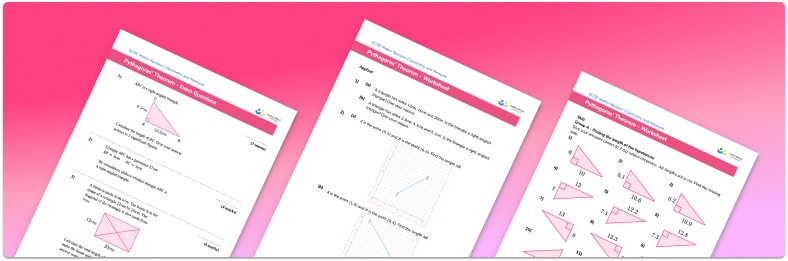# Pythagoras Worksheet• Section 1 of the Pythagoras Theorem worksheet contains 36 skills-based Pythagoras Theorem questions, in 3 groups to support differentiation
• Section 2 contains 4 applied Pythagoras Theorem questions with a mix of worded problems and deeper problem solving questions
• Section 3 contains 3 foundation and higher level GCSE exam questions on Pythagoras Theorem
• Answers and a mark scheme for all Pythagoras Theorem questions are provided
• Questions follow variation theory with plenty of opportunities for students to work independently at their own level
• All questions created by fully qualified expert secondary maths teachers
• Suitable for GCSE maths revision for AQA, OCR and Edexcel exam boards

• This field is for validation purposes and should be left unchanged.

You can unsubscribe at any time (each email we send will contain an easy way to unsubscribe). To find out more about how we use your data, see our privacy policy.

### Pythagoras Theorem at a glance

Pythagoras Theorem states that, in a right angled triangle abc, the square of the length of the hypotenuse (or longest side) c is equal to the sum of the squares of the lengths of the two shorter sides of the triangle, a and b. Its algebraic formula is a2+b2=c2. It is sometimes called the Pythagorean Theorem.

This result can be used to find the length of a missing side of a right angle triangle when the other two are known.

To find the length of the hypotenuse, square the lengths of the sides a and b, and add them. Finally, square root to find the length of the unknown side. To find the length of a missing shorter side (say a), square b and c. We then proceed by subtracting the square of b from the square of c, and taking the square root as before.

Square rooting will often result in non-terminating decimals so students should give their final answers to an appropriate number of decimal places or significant figures.

A Pythagorean triple is a set of three integers that satisfies Pythagoras theorem – for example, 3, 4, 5 or 6, 8, 10. It is useful for students to be able to spot these.

There are some common real life applications of Pythagoras that come up in exam questions, such as finding how far up a wall a ladder reaches.

Looking forward, students can then progress to additional geometry worksheets, for example a or anFor more teaching and learning support on Geometry our GCSE maths lessons provide step by step support for all GCSE maths concepts.

## Do you have KS4 students who need more focused attention to succeed at GCSE?There will be students in your class who require individual attention to help them succeed in their maths GCSEs. In a class of 30, it’s not always easy to provide.

Help your students feel confident with exam-style questions and the strategies they’ll need to answer them correctly with our dedicated GCSE maths revision programme.

Lessons are selected to provide support where each student needs it most, and specially-trained GCSE maths tutors adapt the pitch and pace of each lesson. This ensures a personalised revision programme that raises grades and boosts confidence.

Find out more